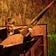# Why You’re Likely To Solve Wordle (according to 100,000 Simulations) | by Daniel Tompkins | Jan, 2022

## tl; DR: If you follow every hint you’ll always be left with only one word by your sixth guess…based on my 100,000 computer simulations`import numpy as npwith open("wordlist_guesses.txt", 'r') as f:all_words = f.readlines()`
`def score_word(chosen, guess):score = [-1, -1, -1, -1, -1]for i, w in enumerate(guess):if w in chosen:if w == chosen[i]:score[i] = 1else:score[i] = 0return score`
`def remove_words(score, guess, word_list):to_remove = []for i, word in enumerate(word_list):for s, w1, w2 in zip(score, word, guess):if s == 1:if w1 != w2:to_remove.append(word)breakelif s == -1:if w2 in word:to_remove.append(word)breakelif s == 0:if w1 == w2:to_remove.append(word)breakelif w2 not in word:to_remove.append(word)breakword_list = list(set(word_list) - set(to_remove))return word_list`
`def wordle(word_list):count = 0chosen_word = np.random.choice(word_list)score = [-1, -1, -1, -1, -1]left = Nonewhile score != [1, 1, 1, 1, 1]:count += 1guess = np.random.choice(word_list)score = score_word(chosen_word, guess)if score == [1, 1, 1, 1, 1]:left = len(word_list)else:word_list = remove_words(score, guess, word_list)return count, left`